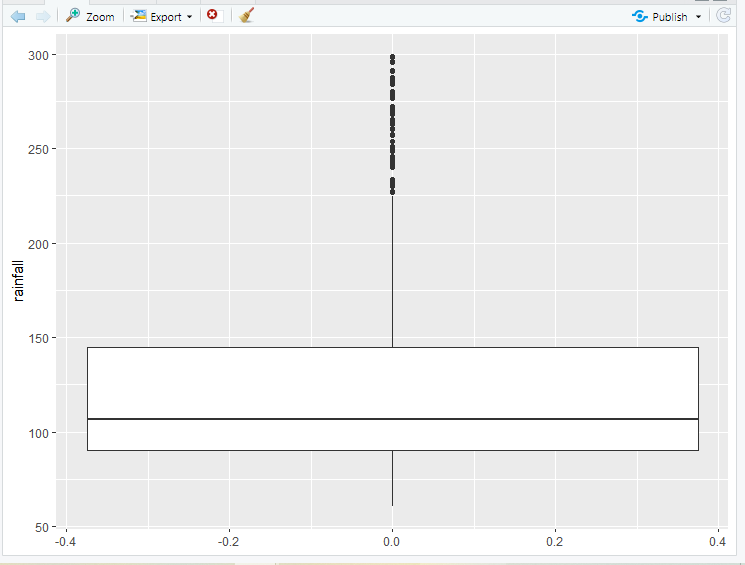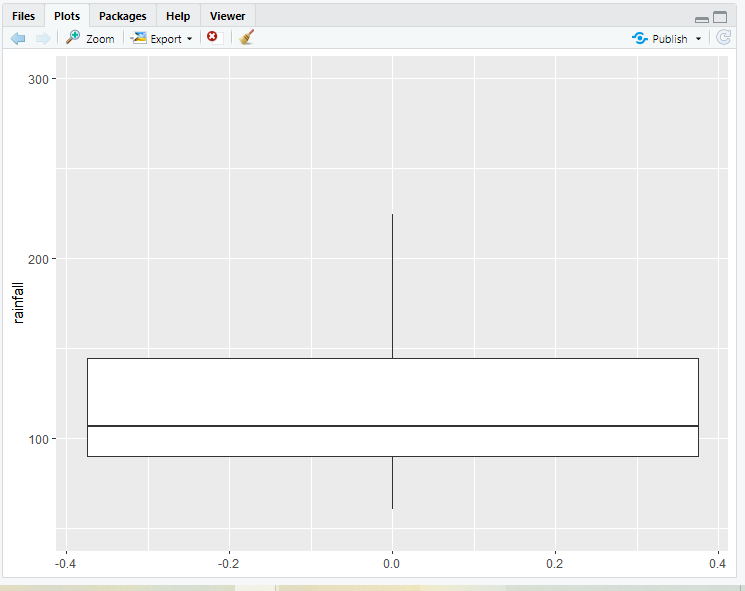Open in App
Not now

# Ignore Outliers in ggplot2 Boxplot in R

• Difficulty Level : Hard
• Last Updated : 30 Jun, 2021

In this article, we will understand how we can ignore or remove outliers in ggplot2 Boxplot in R programming language.

Removing/ ignoring outliers is generally not a good idea because highlighting outliers is generally one of the advantages of using box plots. However, sometimes extreme outliers, on the other hand, can alter the size and obscure other characteristics of a box plot, therefore it’s best to leave them out in those circumstances. We can remove outliers in R by setting the outlier.shape argument to NA. In addition, the coord_cartesian() function will be used to reject all outliers that exceed or below a given quartile. The y-axis of ggplot2 is not automatically adjusted. You can adjust the axis by using the coord_cartesian() function.

For creating Boxplot with outliers we require two functions one is ggplot() and the other is geom_boxplot()

Dataset Used: Crop_recommendation

Let us first create a regular boxplot, without removing any outliers so that the difference becomes apparent.

Example:

## R

 `# Loading``library``(ggplot2)`` ` `# loading data set and storing it in ds variable``ds <- ``read.csv``(``"c://crop//archive//Crop_recommendation.csv"``, header = ``TRUE``)``ds`` ` `# create a boxplot by using geom_boxplot() ``# function of ggplot2 package with outliers``box_plot_crop<-``ggplot``(data=ds, ``aes``( y=rainfall))`` ` `box_plot_crop+``geom_boxplot``()`

Output:Now, for removing the outliers, you can use the outlier.shape to NA argument.

Syntax:

geom_boxplot(outlier.shape = NA)

You can change the axis directly with the coord_cartesian() function since ggplot2 does not automatically adjust the axes. In the coord_catesian() you can set the limit of the axes by using the argument ylim or xlim.

Syntax:

coord_cartesian( xlim = NULL, ylim = NULL, expand = TRUE, default = FALSE, clip = “on )

Parameters:

• xlim, ylim-> set the limits of x and y-axis and also allows zooming in and zoom out.
• expand–  It is TRUE by default, and if it is TRUE then it increases the limit by a small amount to ensure that data and axes do not overlap. and if it is FALSE then the limit is taken from the exact data or the xlim/ ylim.
• default–  used for checking is this is the default coordinate system
• clip- It checks Should the drawing be cropped to fit the plot panel

Example:

## R

 `# Loading``library``(ggplot2)`` ` `# loading data set and storing it in ds variable``ds <- ``read.csv``(``"c://crop//archive//Crop_recommendation.csv"``, header = ``TRUE``)``ds`` ` `# remove outliers or create boxplot without outliers``box_plot_crop<-``ggplot``(data=ds, ``aes``(y = rainfall)) ``box_plot_crop+ ``geom_boxplot``(outlier.shape = ``NA``) +``  ``coord_cartesian``(ylim =  ``c``(50, 300))`

Output:My Personal Notes arrow_drop_up